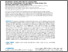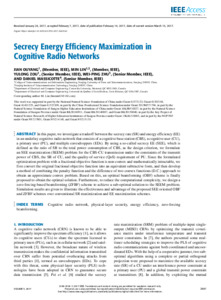RECHERCHER

# Secrecy energy efficiency maximization in cognitive radio networks

### Téléchargements

Téléchargements par mois depuis la dernière année

Plus de statistiques...

Ouyang, J., Lin, M., Zou, Y., Zhu, W. P. et Massicotte, D. (2017). Secrecy energy efficiency maximization in cognitive radio networks. IEEE Access, 5 . p. 2641-2650. ISSN 2169-3536 DOI 10.1109/ACCESS.2017.2667882Prévisualisation PDF Télécharger (7MB) | Prévisualisation

## Résumé

In this paper, we investigate a tradeoff between the secrecy rate (SR) and energy efficiency (EE) in an underlay cognitive radio network that consists of a cognitive base station (CBS), a cognitive user (CU), a primary user (PU), and multiple eavesdroppers (EDs). By using a so-called secrecy EE (SEE), which is defined as the ratio of SR to the total power consumption of CBS, as the design criterion, we formulate an SEE maximization (SEEM) problem for the CBS-CU transmission under the constraints of the transmit power of CBS, the SR of CU, and the quality-of-service (QoS) requirement of PU. Since the formulated optimization problem with a fractional objective function is non-convex and mathematically intractable, we first convert the original fractional objective function into an equivalent subtractive form, and then develop a method of combining the penalty function and the difference of two-convex functions (D.C.) approach to obtain an approximate convex problem. Based on this, an optimal beamforming (OBF) scheme is finally proposed to obtain the optimal solution. Furthermore, to reduce the computational complexity, we design a zero-forcing-based beamforming (ZFBF) scheme to achieve a sub-optimal solution to the SEEM problem. Simulation results are given to illustrate the effectiveness and advantage of the proposed SEE oriented OBF and ZFBF schemes over conventional SR maximization and EE maximization schemes.

Type de document: Article Cognitive radio network Physical-layer security Energy efficiency Zero-forcing beamforming 09 mai 2022 14:15 09 mai 2022 14:15 Version officielle de l'éditeur https://depot-e.uqtr.ca/id/eprint/10152

#### Actions (administrateurs uniquement)Éditer la notice